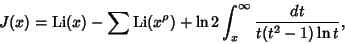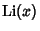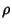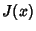## Riemann's Formulawhereis the Logarithmic Integral, the sum is taken over all nontrivial zeros(i.e., those other than,, ...) of the Riemann Zeta Function, andis Riemann Weighted Prime-Power Counting Function.

See also Logarithmic Integral, Prime Number Theorem, Riemann Weighted Prime-Power Counting Function, Riemann Zeta Function